Ch 1 angle review
If <BOC = 40, find the measure of <COD. *
1 point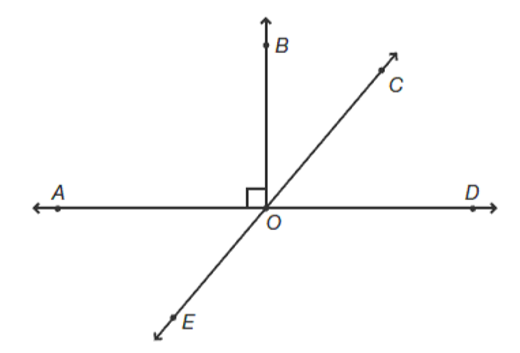Find the measure of <CGB *
1 point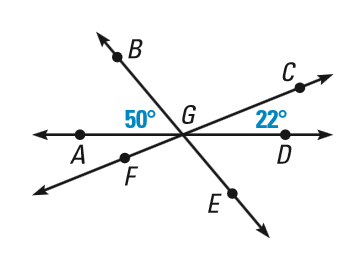ACT example *
1 point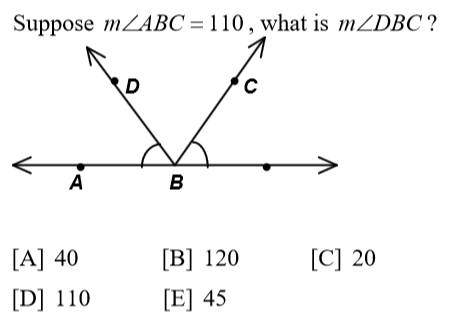Find angle x *
1 point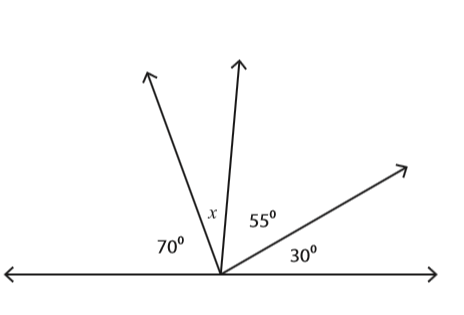Find the measure of <FGD. *
1 pointLast Name *
Solve for x. *
1 point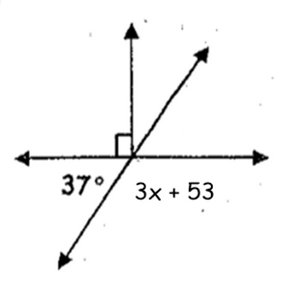ACT examples *
1 point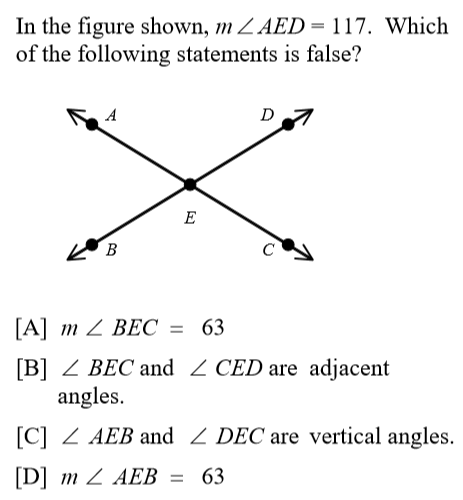If <EOB = 132, What is the measure of <COB? *
1 pointFind angle x *
1 point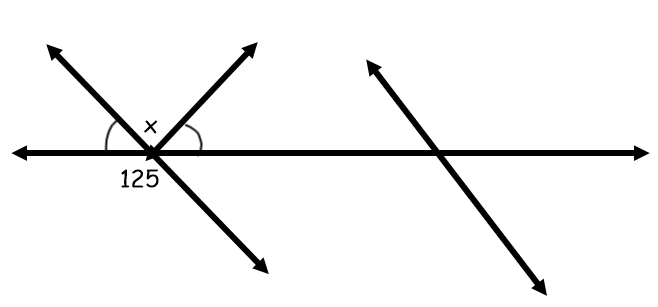Period *
Find the measure of <AGF *
1 pointNever submit passwords through Google Forms.
This content is neither created nor endorsed by Google. Report Abuse - Terms of Service - Privacy Policy